Follow

# Guide to: Text Annotation Test Questions

## Overview

Test Questions are rows with known answers (also known as golden answers). They are randomly inserted throughout the job with the purpose of measuring the accuracy of judgments submitted by contributors.

## Enable Nested Spans

The checkbox "Enable nested spans" (or attribute `allow-nesting="true"` in CML) on the design page impacts how test questions are configured. The main difference is that:

1. If nested spans are not enabled (default), giving a string multiple layers of annotation is regarded as multiple acceptable answers.
2. If nested spans are enabled, giving a string multiple layers of annotation is regarded as the only correct way to annotate this string.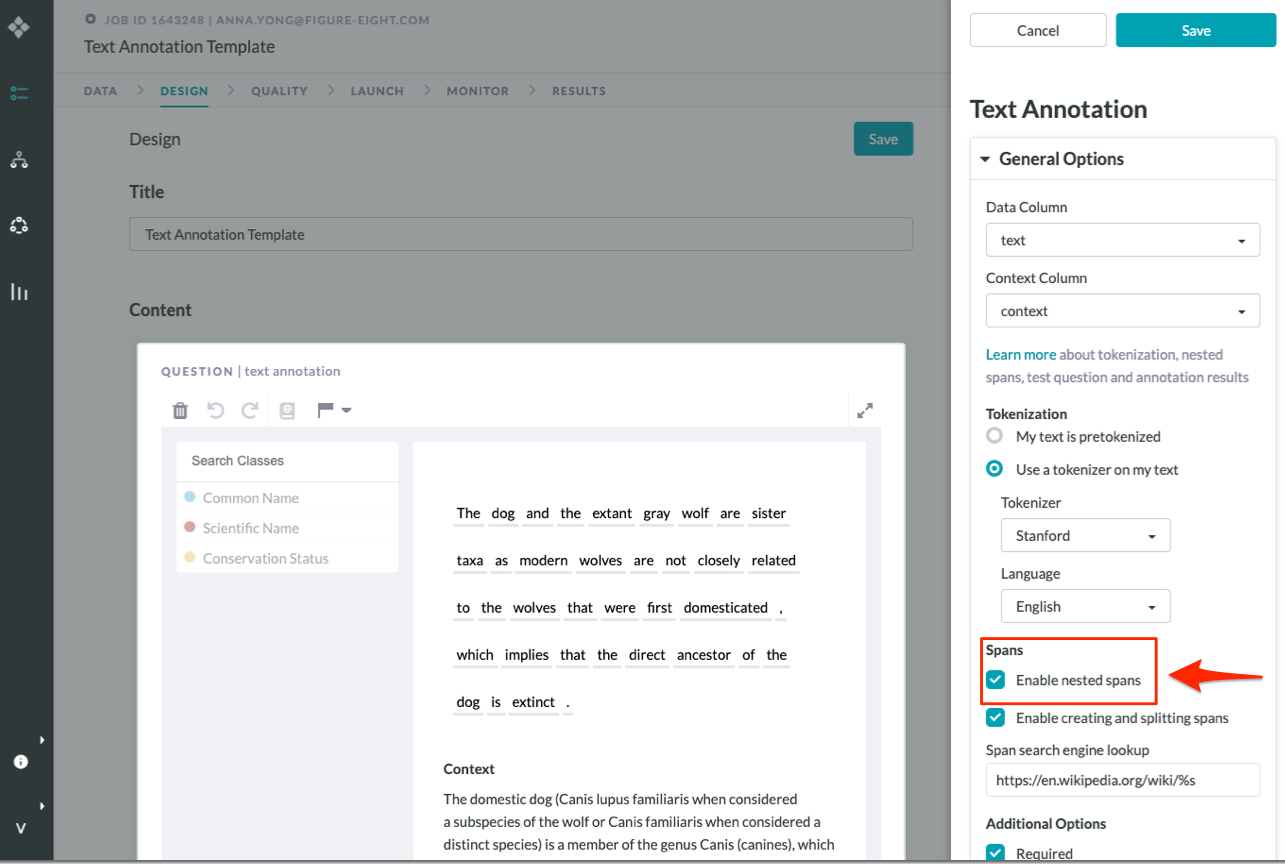Fig. 1: Enable nested spans checkbox on Design page

### For both scenarios:

• You have the flexibility to set a passing threshold percentage for each test question.
• Scoring above this percentage would be regarded as pass.
• A contributor's judgment accuracy is calculated this way:
• Number of correctly annotated spans / (number of golden spans + number of incorrectly annotated spans)

### In the case where nested spans are not enabled:

• This creates multiple correct ways of annotating a string.
• The tool calculates the accuracy score against all annotation possibilities, then take the highest score as the final accuracy.
• For each test question you create, you can set a passing accuracy threshold from 1% to 100%.
• Scoring higher than this threshold would allow contributors' answer to pass.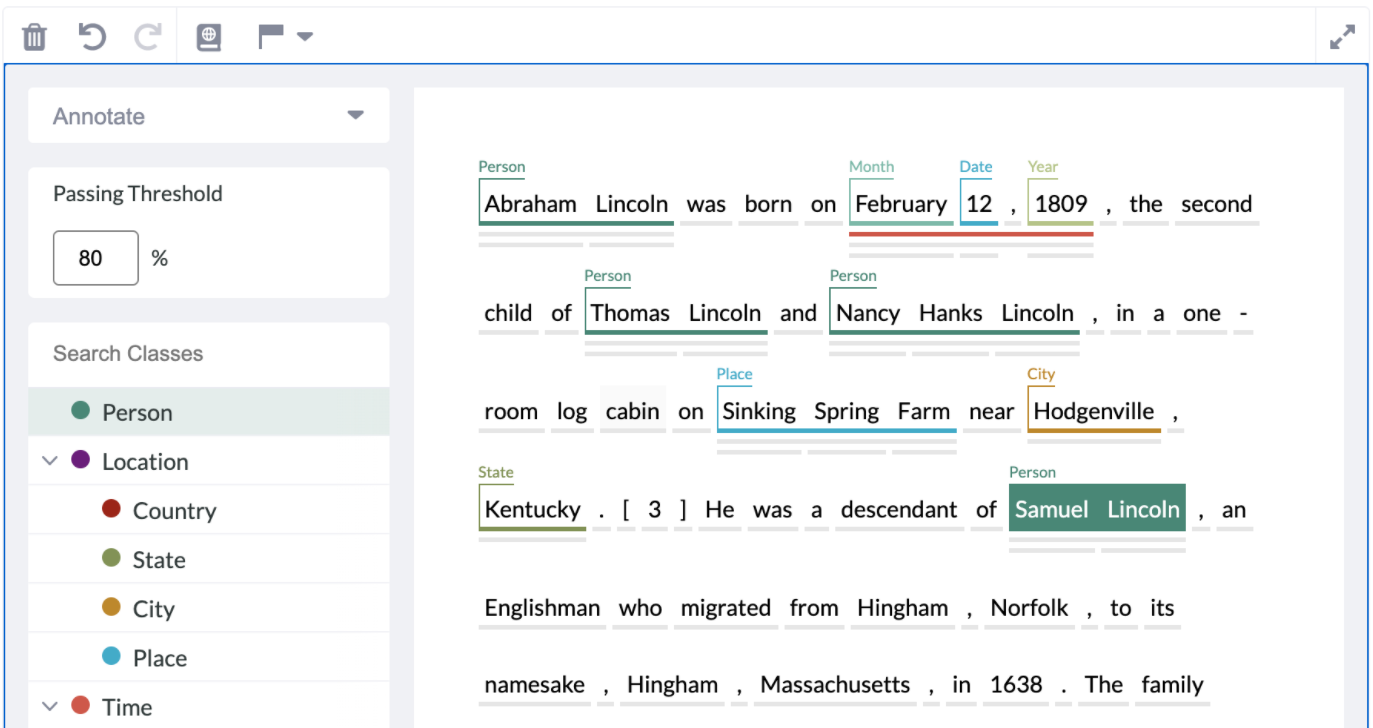Fig. 2: Test Question Creation Page with Nested Spans

## Examples

#### Example 1 - Nested spans not enabled

 Test Question Golden Answer: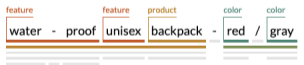Possibility 1: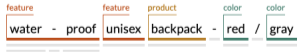Possibility 2: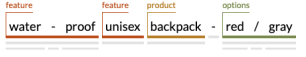Possibility 3: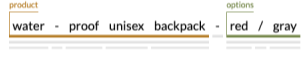Possibility 4: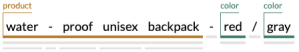Matching any of the possibilities above would mean an 100% accuracy. Below are some examples that are not 100% correct.

 Answer 1 - 67% Accuracy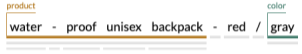Reason: this answer is closest to possibility #4, with 2 correct spans and 0 incorrect spans. Therefore the accuracy would be 2/(3+0) = 67% Answer 2 - 33% Accuracy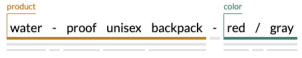Reason: this answer is closest to possibility #3 and #4. Measuring against #3 would give you the accuracy of 1/(2+1) = 33%. With #4, the accuracy would be 1/(3+1) = 25%. In this case, we’ll take the higher score to be the accuracy.

#### Example 2 - Nested spans enabled

 Test Question golden answer: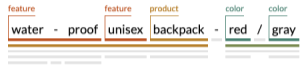Answer 1 - 29% Accuracy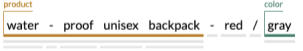Reason: this answer contains 2 correct spans and 0 incorrect spans, so that accuracy would be 2/(7+0) = 29% Answer 2 – 67% Accuracy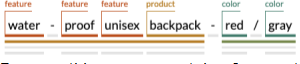Reason: this answer contains 6 correct spans and 2 incorrect spans, so the accuracy would be 6/(7+2) = 67%